• SVC多分类
千次阅读
2021-02-03 07:49:08

SVM可以用于分类、回归、异常检测。SVM库中包括SVC、LinearSVC接口

1.导入svm库

from sklearn import svm

2.制作训练集和测试集

x, y = np.split(data, (4,), axis=1)

x = x[:, :2]

x_train, x_test, y_train, y_test = train_test_split(x, y, random_state=1, train_size=0.6)

split(数据，分割位置，轴=1(水平分割) or 0(垂直分割))。

x = x[:, :2]是为方便后期画图更直观，故只取了前两列特征值向量训练

sklearn.model_selection.train_test_split随机划分训练集和测试集。

3.训练SVM

clf = svm.SVC(C=0.8, kernel='rbf', gamma=20, decision_function_shape='ovr')

clf.fit(x_train, y_train.ravel())

C:惩罚参数

默认值为1，惩罚参数是由于松弛变量而加入的，它表征的是对错误分类的惩罚程度，也就是不允许分类出错的程度。

C越大，表明越不允许分类出错，但是C越大越可能过拟合。

C太小的话趋于0的话，分类将不会关注分类是否正确的问题，只要求间隔 越大越好，此时分类也没有意义。

Kernel:核函数

作用：核函数的引入是为了解决线性不可分的问题，讲分类点映射的高维空间中以后，转化为可线性分割的问题。

kernel=‘linear’时，为线性核，C越大分类效果越好，但可能会过拟合；

kernel='rbf'时，为高斯核，gamma值越小，分类界面越连续；gamma值越大，分类界面越“散”，分类效果越好，但可能会过拟合；

kernel='poly'：多项式核

kernel=sigmoid’：Sigmoid核函数

decision_function_shape参数

decision_function_shape='ovr'时，为one v rest,即一个类别与其他ov类别进行划分；

decision_function_shape='ovo'时，为one v one,即将类别两两进行划分，用二分类的方法模拟多分类的结果；

4.计算SVC分类器的准确率

print clf.score(x_train, y_train) # 精度

y_hat = clf.predict(x_train)

show_accuracy(y_hat, y_train, '训练集')

print clf.score(x_test, y_test)

y_hat = clf.predict(x_test)

show_accuracy(y_hat, y_test, '测试集')

如果想查看决策函数，可以通过decision_function()实现

print 'decision_function:\n', clf.decision_function(x_train)

print '\npredict:\n', clf.predict(x_train)

更多相关内容
• train, y_test = train_test_split(X,y, test_size=0.3,random_state=100) model = SVC(C=1, kernel='rbf', gamma=1.0, decision_function_shape="ovr") model.fit(X=X_train,y=y_train) dtb = model.decision_...
import xlwt
import numpy as np
import pandas as pd
from copy import deepcopy
from sklearn.metrics import accuracy_score, mean_absolute_error, f1_score, recall_score
from collections import OrderedDict
from sklearn.linear_model import LogisticRegression
from sklearn.model_selection import StratifiedKFold
from pathlib import Path
import matplotlib.pyplot as plt
from scipy.spatial.distance import pdist, squareform
from sklearn.svm import SVC
from sklearn.model_selection import train_test_split
from sklearn import datasets

if __name__ == '__main__':
labels = np.sort(np.unique(y))
nClass = len(labels)
X_train,X_test, y_train, y_test = train_test_split(X,y, test_size=0.3,random_state=100)

model = SVC(C=1, kernel='rbf', gamma=1.0, decision_function_shape="ovr")
model.fit(X=X_train,y=y_train)
dtb = model.decision_function(X=X_test)
y_pred = model.predict(X=X_test)
print(dtb)
print("精度：",accuracy_score(y_true=y_test,y_pred=y_pred))

print("=============================================")

model_list = OrderedDict()
dist_matrix = np.zeros((len(y_test),nClass))
for tar in labels:
target = np.zeros(len(y_train))
for i, lab in enumerate(y_train):
if lab == tar:
target[i] = 1
else:
target[i] = 0
model_list[tar] = SVC(C=1, kernel='rbf', gamma=1.0, decision_function_shape="ovo")
model_list[tar].fit(X=X_train,y=target)
dist_matrix[:,tar] = model_list[tar].decision_function(X_test)
y_pred_2 = []
for i in range(len(y_test)):
print(dist_matrix[i])
print(y_test[i])
y_pred_2.append(np.argmax(dist_matrix[i]))

print("精度====",accuracy_score(y_true=y_test,y_pred=y_pred_2))

中间过程不同，但结果相同

展开全文• 本文提出了一种面向SVC的快速模式决策算法，该方法首先利用模式在层间、帧间和空间的相关性，决定当前宏块的起始搜索模式，然后利用相邻运动向量的差异性决定是否合并或拆分当前分块进行细化搜索。从测试结果来看...可伸缩视频编码
• 一种支持向量机分类算法的应用，主要用于数据的分类运算。
• svm.SVC 支持向量机分类是一个很有效的分类方式，但是其只对2分类有效，不过，可以将多分类经过次2分类最终实现多分类，而sklearn中的multiclass包就可以实现这种方式，减少我们重复造轮子。 import numpy as np ...

### github地址

svm.SVC 支持向量机分类是一个很有效的分类方式，但是其只对2分类有效(sklearn中并不是，针对多分类其使用了1vs多，decision_function_shape : 'ovo', 'ovr', default='ovr', 这里假装只对2分类有效，用来进行下面的内容， ￣□￣｜｜），不过，可以将多分类经过多次2分类最终实现多分类，而sklearn中的multiclass包就可以实现这种方式，减少我们重复造轮子。

import numpy as np
from sklearn.datasets import load_digits
from sklearn.preprocessing import label_binarize
from sklearn.multiclass import OneVsRestClassifier
from sklearn.model_selection import train_test_split

x, y = digits.data, digits.target
y = label_binarize(y, classes=list(range(10)))
x_train, x_test, y_train, y_test = train_test_split(x, y)
model = OneVsRestClassifier(svm.SVC(kernel='linear'))
clf = model.fit(x_train, y_train)

In : clf.score(x_train, y_train)
Out: 0.97475872308834444

In : clf.score(x_test, y_test)
Out: 0.85999999999999999

In : np.argmax(y_test, axis=1)
Out: array([0, 0, 2, ..., 5, 6, 7], dtype=int64)

In : np.argmax(clf.decision_function(x_test), axis=1)
Out: array([0, 0, 2, ..., 5, 6, 7], dtype=int64)


### github地址

展开全文sklearn svc多分类 binarize
• 分类作为数据挖掘领域中一项非常重要的任务，它的目的是学会一个分类函数或分类模型(或者叫做分类器)，而支持向量机本身便是一种监督式学习的方法
• ## SVM-SVC分类

万次阅读 2018-12-06 01:21:09
用于二元和多元分类器、回归和新奇性检测 良好的预测生成器，提供了鲁棒的过拟合、噪声数据和异常点处理 成功处理了涉及到很变量的场景 当变量比样本还是依旧有效 快速，即使样本量大于1万 自动检测数据的...

SVM优点：

1. 用于二元和多元分类器、回归和新奇性检测
2. 良好的预测生成器，提供了鲁棒的过拟合、噪声数据和异常点处理
3. 成功处理了涉及到很多变量的场景
4. 当变量比样本还多是依旧有效
5. 快速，即使样本量大于1万
6. 自动检测数据的非线性，不用做变量变换

SVM缺点：

1. 应用在二元分类表现最好，其他预测问题表现不是太好
2. 变量比样例多很多的时候，有效性降低，需要使用其他方案，例如SGD方案
3. 只提供预测结果，如果想要获取预测概率，需要额外方法去获取
4. 如果想要最优结果，需要调参。

使用SVM预测模型的通用步骤

1. 选择使用的SVM类
2. 用数据训练模型
3. 检查验证误差并作为基准线
4. 为SVM参数尝试不同的值
5. 检查验证误差是否改进
6. 再次使用最优参数的数据来训练模型

SVM种类，用途和关键参数表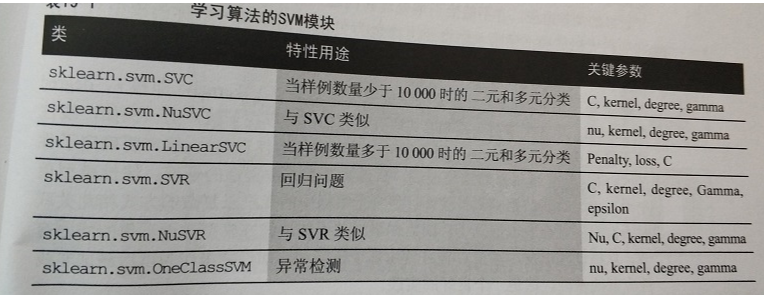主要分为三类：1、分类 2、回归 3、异常检测

TIPs:SVM模块包含2个库：libsvm和liblinear,拟合模型时，python和这两个库有数据流，会消耗一部分内存。

如果内存足够，最好能够把SVM的cashe_size参数设置大于200M，例如1000M。

参数问题：

• C参数表明算法要训练数据点需要作出多少调整适应，C小，SVM对数据点调整少，多半取平均，仅用少量的数据点和可用变量，C值增大，会使学习过程遵循大部分的可用训练数据点并牵涉较多变量。C值过大，有过拟合问题，C值过小，预测粗糙，不准确。
• kernel
• degree
• gamma

一、SVC分类（手写字体识别问题）

#载入数据
from sklearn import datasets
X,y = digits.data, digits.target
#画图展示
import matplotlib.pyplot as plt

#此处注意，k,img代表的数字在1-10之间，故enmerate（）需要增加一个start=1的参数
for k,img in enumerate(range(10),start=1):
plt.subplot(2, 5, k)
plt.imshow(digits.images[img],cmap='binary',interpolation='none')
plt.show()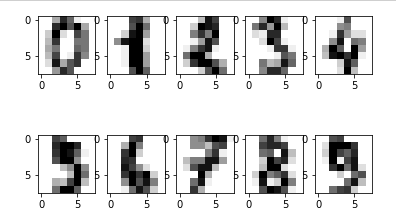print(X.shape)
#X
from scipy.stats import itemfreq
print(itemfreq(y))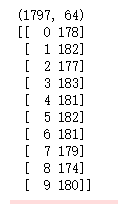TIPs如果遇到类不平衡的问题：

1、保持不平衡，偏向于评率高的类做预测

2、使用权重在类间建立平等，允许对观察点多次计数

3、删减拥有过多样例的勒种的一些样例

解决类不平衡问题的参数方法：

• sklearn.svm.SVC中的class_weight='auto'
• fit中的sample_weight

#数据区分测试集和训练集，并将数据标准化
from sklearn.cross_validation import train_test_split, cross_val_score
from sklearn.preprocessing import MinMaxScaler
# We keep 30% random examples for test
X_train, X_test, y_train, y_test = train_test_split(X, y, test_size=0.3, random_state=101)
# We scale the data in the range [-1,1]
scaling = MinMaxScaler(feature_range=(-1, 1)).fit(X_train)
X_train = scaling.transform(X_train)
X_test  = scaling.transform(X_test)

from sklearn.svm import SVC
# We balance the clasess so you can see how it works
learning_algo = SVC(kernel='linear', class_weight='balanced')
cv_performance = cross_val_score(learning_algo, X_train, y_train, cv=5)
test_performance = learning_algo.fit(X_train, y_train).score(X_test, y_test)
print ('Cross-validation accuracy score: %0.3f, test accuracy score: %0.3f' % (np.mean(cv_performance),test_performance))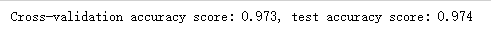数据结果显示精度很好

C参数默认1，下面使用网格搜索来选择最优的C参数

from sklearn.grid_search import GridSearchCV
learning_algo = SVC(class_weight='balanced', random_state=101)

#定义了C的一个列表，包含7个值，从10^-3到10^3
search_space = {'C': np.logspace(-3, 3, 7)}
gridsearch = GridSearchCV(learning_algo, param_grid=search_space, scoring='accuracy', refit=True, cv=10, n_jobs=-1)
gridsearch.fit(X_train,y_train)
cv_performance = gridsearch.best_score_
test_performance = gridsearch.score(X_test, y_test)
print ('Cross-validation accuracy score: %0.3f, test accuracy score: %0.3f' % (cv_performance,test_performance))
print ('Best C parameter: %0.1f' % gridsearch.best_params_['C'])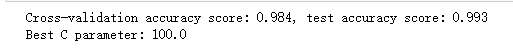C取值为100的时候，模型最优。

展开全文• svm.SVC参数 文档 sklearn.svm.SVC(C=1.0, kernel='rbf', degree=3, gamma='auto', coef0=0.0, shrinking=True, probability=False, tol=0.001, cache_size=200, class_weight=None, verbose=False, max_iter=-1, ...
• 二元分类器在两个类中区分，而分类器（也称为分类器）可以区分两个以上的类。有一些算法（如随机森林分类器或朴素贝叶斯分类器）可以直接处理个类。也有一些严格的二元分类器（如支持向量机分类器或线性...sklearn 多分类
• svm_nu 分类源码 用于svm的分类编程同svm_c 相近
• ## Sklearn实现SVC

千次阅读 2021-09-28 22:00:17
官方网站：sklearn关于支持向量机多分类的介绍 sklearn.svm中的模型是基于libsvm和liblinear两个包开发出来的。它提供了一系列的支持向量机算法，包括分类SVC, Support Vector Classifier）、回归（SVR, Support ...sklearn 支持向量机 机器学习
• ONE_CLASS =102, EPS_SVR =103, NU_SVR =104 } 一般我们使用SVM进行二分类或者多分类任务，选择第一种SVM::C_SVC即可。 还有一个函数就是： CV_WRAP virtual void setKernel(int kernelType) = 0; 这个函数用于设置...
• 混淆矩阵是二分类问题的多维衡量指标体系，在样本不平衡时极其有用。在混淆矩阵中，我们将少数类认为是正 例，多数类认为是负例。在决策树，随机森林这些普通的分类算法里，即是说少数类是1，多数类是0。在SVM里， ...sklearn 支持向量机
• 其实sklearn中产生数据、训练数据、预测结果都非常的简单。 就是打印这个决策边界有些麻烦...from sklearn.svm import SVC from sklearn.svm import LinearSVC from sklearn.svm import NuSVC from sklearn import ...sklearn python 机器学习
• API说明： 中文：http://sklearn.apachecn.org/cn/0.19.0/modules/svm.html 英文：... API使用：（SVC）（Support Vector Classification.） from sklearn import svm X = [[0...
• Classify：SVC、nuSVC、LinearSVC Regression：SVR、nuSVR、LinearSVR OneClassSVM 本文采用Classify系列，classify三个模型的区别；参数详解 预处理 import pandas as pd path = "../Data/classify.csv" rawdata = ...机器学习 支持向量机 svm python
• A,B,C这三条直线，都可以把红蓝球给分开。究竟哪一个最好呢？ 相比于直线 A 和直线 B，直线 C 的划分更优，容错...转动这个最优决策面，你会发现可能存在个最优决策面，它们都能把数据集正确分开，这些最优决策面..机器学习 人工智能
• 对于这个点已经介绍的非常了，不管是西瓜书还是各种博客，就是我们需要找到一个超平面，用这个超平面把数据划分成两个类别，最开始的SVM就是在二分类的问题上应用，在之后被扩展到类别的分类。对于SVM的推导公式...机器学习 python 支持向量机
• 回归预测 | MATLAB实现SVC(支持向量机)多分类预测 目录回归预测 | MATLAB实现SVC(支持向量机)多分类预测基本介绍环境配置程序设计参考资料致谢 基本介绍 支持向量机的基础概念可以通过一个简单的例子来解释。让我们...机器学习 SVM 多分类
• ## Svm实现多分类

万次阅读 多人点赞 2019-05-14 17:17:23
Svm实现多分类Svm实现分类原理代码实现训练的图片 Svm实现分类原理 1.支持向量机分类算法最初只用于解决二分类问题，缺乏处理多分类问题的能力。后来随着需求的变化，需要svm处理多分类分为。目前构造多分类...python svm 机器学习
• import numpy as np import matplotlib.pyplot as plt from sklearn.svm import SVC class MySVM: X=[];y=[] m=0;n=0 fignum=1 def __init__(self,params={'kernel': 'linear'}): self.modu...SVM
• 根据迁移学习思想, 针对分类问题, 以支持向量机(SVM) 模型为基础提出一种新的迁移学习分类算法CC-TSVM. 该方法以邻域间的分类超平面为纽带实现源域对目标域的迁移学习. 具体地, 以支持向量分类的约束条件完成对目标...
• ## sklearn学习笔记（3）svm多分类

万次阅读 多人点赞 2017-06-06 19:55:45
SVC、NuSVC、LinearSVC都可以在一个数据集上实现多分类SVC和NuSVC方法类似，但是有不同的输入参数集和不同的数学表述。另一方面，linearSVC是SVC的在线性核的基础上的另一种实现，所以LinearSVC不能不能接受...svm
• svm工具箱，应用于matlab平台，给出了C_SVC二类分类算法的历程
• SVM具有分类功能(SVC，C是Classification(分类)的首字母); 也具有回归功能(SVR，R是Regression(回归)的首字母)。 [问题分析] 在[Python数据挖掘] sklearn-KMeans聚类一文中，实现了对以下数据集的聚类： YZN,...sklearn 分类
• 支持向量分类-支持向量归纳SVC-SVR实例，适合于初学者...